# Python Drawing: Intro to Python Matplotlib for Data Visualization (Part 1)Want to know how Python is used for plotting and data visualization? Interested in learning one of the most commonly used data visualization libraries in Python? If so, you’re in the right place.

In this installment of a two-part tutorial, we’ll learn how to use matplotlib, one of the most commonly used data visualization libraries in Python. Over the course of both articles, we’ll create different types of graphs, including:

• Line plots
• Histograms
• Bar plots
• Scatter plots
• Stack plots
• Pie charts

We’ll also see what different functions and modules are available in matplotlib.

Here, we’ll explore how to create just line plots and histograms with matplotlib. In addition to plotting graphs, we’ll also see how to change the default size of graphs and how to add labels, titles, and legends to their axes.

### Installing the Matplotlib Library

The simplest way to install matplotlib is to use the pip installer, which comes with most standard Python installations. Execute the following command from your preferred terminal:

```pip install matplotlib
```

If you’re using the Anaconda distribution of Python, you can also use the commands mentioned in the official Anaconda documentation to install the matplotlib library.

### Importing Required Libraries: Numpy and MatplotLib.pyplot

Once we’ve installed matplotlib, the next step is to import the required libraries. The pyplot library of matplotlib is used to plot different types of graphs. We’ll import it along with the numpy library.

You’ll see how exactly we can use these two libraries in a later section. For now, execute the following script to import them:

```import matplotlib.pyplot as plt
%matplotlib inline
import numpy as np
```

Since I’m using Jupyter Notebook to execute the scripts in this article, I have to execute the statement `%matplotlib` inline, which tells the IDE to plot the graphs within its interface. If you’re not using an IDE like this, you don’t need to execute this statement.

Another important thing to note is that we renamed `pyplot` as `plt` when importing, since it’s easier to type and is a standard nickname for `pyplot`. From now on in this article, we’ll continue using this nickname.

Now, we have everything we need to start plotting different types of matplotlib graphs.

### Changing Plot Size Using pyplot

To see the default plot size of graphs drawn by `plt`, execute the following script:

```plot_size = plt.rcParams["figure.figsize"]
print(plot_size)
print(plot_size)
```

In the script above, we used the `rcParams` attribute of the `plt` module and pass in “`figure.figsize`” as a parameter, which returns a list containing the default width and height of the plot. The first index contains the width, and the second index contains height. Both values are printed to the screen. You’ll see 6 and 4 in the output, which means that the default width of the plot is 6 inches and the default height is 4 inches.

To change the plot size, execute the following script:

```plot_size = 8
plot_size = 6
plt.rcParams["figure.figsize"] = plot_size
```

In the script above, we changed the width and height of the plot to 8 and 6 inches, respectively.

### Line Plots

The line plot is the simplest plot in the matplotlib library; it shows the relationship between the values on the x- and y-axes in the form of a curve.

To create a line plot, you can use the plot function of the `plt` module. The first argument to the `plot` function is the list of values that you want to display on the x-axis. The second argument is the list of values to be drawn on the y-axis. Take a look at the following example:

```plt.plot([-3,-2,-1,0,1,2,3],[9,4,1,0,1,4,9])
plt.show()
```

In the script above, we have six values in the list for the x-axis. On the y-axis, we have the squares of the x values. This means that the line plot will display the square function, as shown in the output. Note that the default plot color for matplotlib graphs is blue.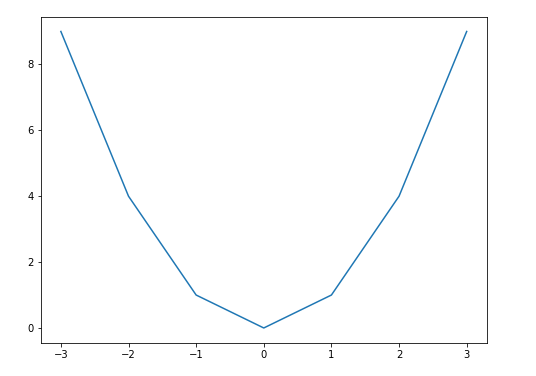It’s important to mention that you need to call the `show` function of the `plt` module if you’re using an editor other than Jupyter Notebook. In Jupyter, the show function is optional.

#### Producing Smooth Curves

Instead of manually entering the values for the lists for the x and y-axis, we can use the linspace function of the numpy library. This function takes three arguments: the lower bound for the values to generate, the upper bound, and the number of equally spaced points to return between the lower and upper bounds. Look at the following script:

```x = np.linspace(-15, 14, 30)
y = np.power(x,3)

plt.plot(x, y, "rebeccapurple")
plt.show()
```

In the above script, we also made use of the power function of the numpy library to calculate the cube of each element in the x array. In the output, you’ll see the line for the cube function displayed in purple, since we specified '`rebeccapurple`' as the third parameter of the `plot` function.

Note for beginners: A function in programming performs specific operations. To pass data to a function, we use arguments. The function then uses the arguments passed to it. For instance, in the plot function, the first parameter is the data to be plotted on the x axis, the second parameter is the data to be plotted on the y axis, and the third parameter is the color code. A color code of ‘`rebeccapurple`’ corresponds to a shade of purple.

Here’s a chart of other colors you can use: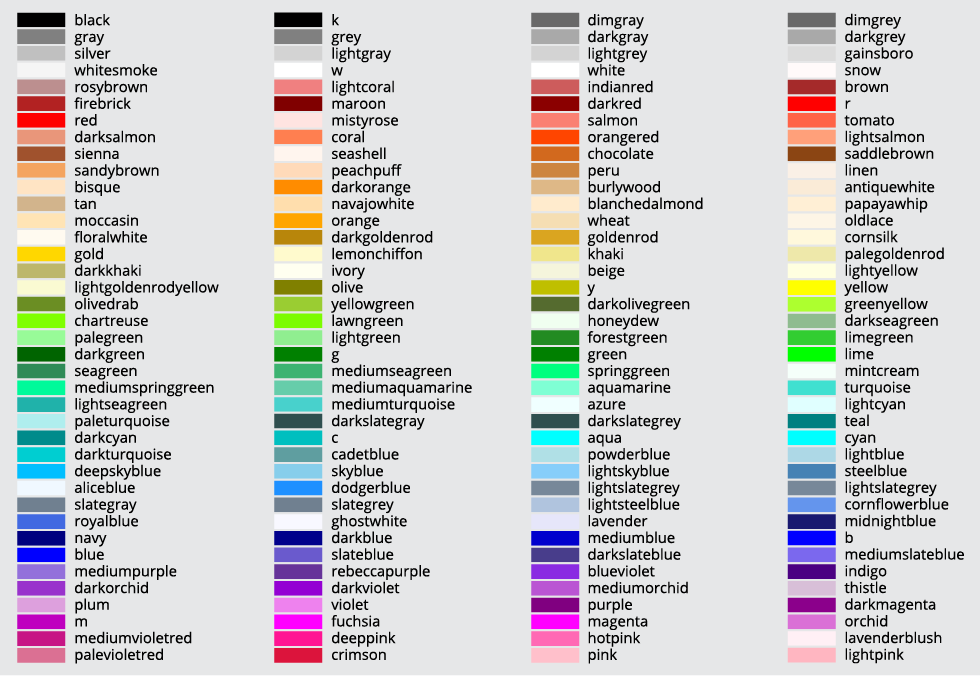The output looks like this:#### Adding Labels, Titles, and Legends

To add labels to the x- and y-axes, you can use the `xlabel` and `ylabel` functions of the `plt` module. Similarly, to add title, you can use `title` function as shown below:

```x = np.linspace(-15, 14, 30)
y = np.power(x,3)

plt.xlabel("input")
plt.ylabel("output")
plt.title("Cube Root")
plt.plot(x, y, "deepskyblue")
plt.show()
```

In the output, you should see your new axis labels and title:To add legends to your plot, you’ll have to pass in a value for the `label` attribute of the plot function as shown below:

```x = np.linspace(-15, 14, 30)

cube = np.power(x,3)
square = np.power(x,2)

plt.xlabel("input")
plt.ylabel("output")
plt.title("Cube Root")

plt.plot(x, cube, "rebeccapurple", label = "Cube")
plt.plot(x, square , "deepskyblue", label = "Square")
plt.legend()
plt.show()
```

In the script above, we have two plots: one for the square function and another for the cube function. To help distinguish the two, we can not only use different colors but also include a legend that clearly labels which is which. In the script above, the legend for the cube plot has been aptly named Cube and will be drawn in purple. The legend for the square plot is named Square and will be drawn in blue. The output of the script above looks like this: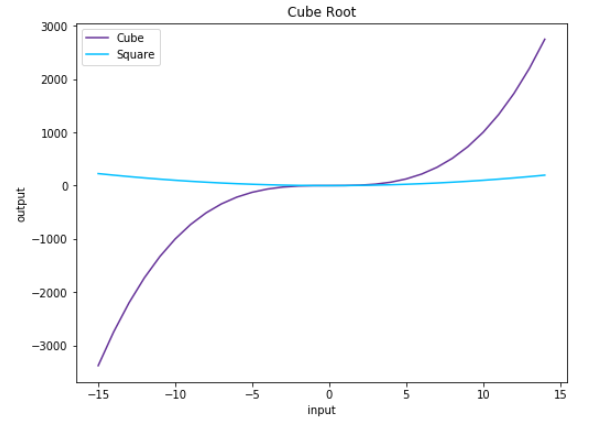#### Pro Tip: How to Improve Matplotlib Line Plots

You can also add markers to the data points on a line plot. To do so, you need to pass a value for the marker parameter of the plot function as shown below:

```x = np.linspace(-15, 14, 30)
x = np.linspace(-15, 14, 30)

cube = np.power(x,3)
square = np.power(x,2)

plt.xlabel("input")
plt.ylabel("output")
plt.title("Cube Root")

plt.plot(x, cube, "rebeccapurple", marker = "o", label = "Cube")
plt.plot(x, square , "deepskyblue", marker = "v", label = "Square")
plt.legend()
plt.show()
```

In the script above, we specified ‘`o`’ as the value for the marker of the cube function; this will generate circles for the data points. Similarly, for the square function, we specified ‘`v`’ as the value for the marker; this uses an upside-down triangle for the points: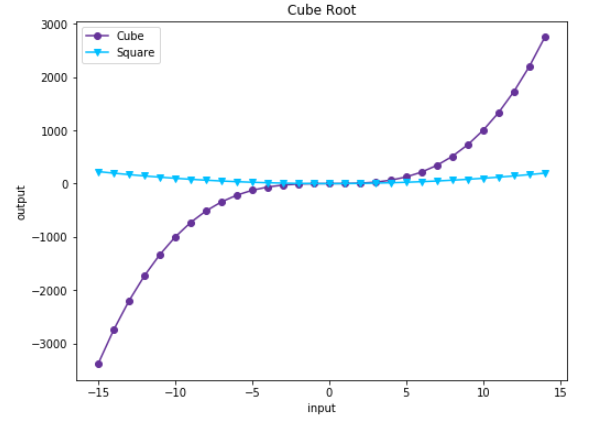The codes to generate different types of markers in matplotlib can be found here.

### Histograms

A histogram shows the distribution of data in the form of data intervals called “bins.” To plot a histogram, you need to call the `hist` function of the `plt` module. The first argument is the data set, the second is the bins, and the third is the type of histogram you want to plot. You can also use the optional `rwidth` argument, which defines the width of each interval or “bin” in the histogram. Look at the following example:

```stock_prices = [23,21,43,32,45,34,56,23,67,89,23,21,43,32,45,34,56,23,67,89,23,21,43,32,45,34,56,23,67,89]

bins = [20,40,60,80,100]

plt.hist(stock_prices, bins, color = "rebeccapurple", histtype="bar", rwidth=0.9)
plt.show()
```

In the script above, we have imaginary data on the average stock prices of thirty companies. We define five bins for the data intervals. Next, we use the `hist` function to plot this data. The output looks like this:You can also create a horizontal histogram. To do so, you simply need to pass in the value ‘`horizontal`’ as the value for the orientation parameter of the `hist` function:

```stock_prices = [23,21,43,32,45,34,56,23,67,89,23,21,43,32,45,34,56,23,67,89,23,21,43,32,45,34,56,23,67,89]

bins = [20,40,60,80,100]

plt.hist(stock_prices, bins, color = "deepskyblue", histtype="bar", rwidth=0.9, orientation = "horizontal")
plt.show()
```
In the output, you’ll see a horizontal histogram as shown below: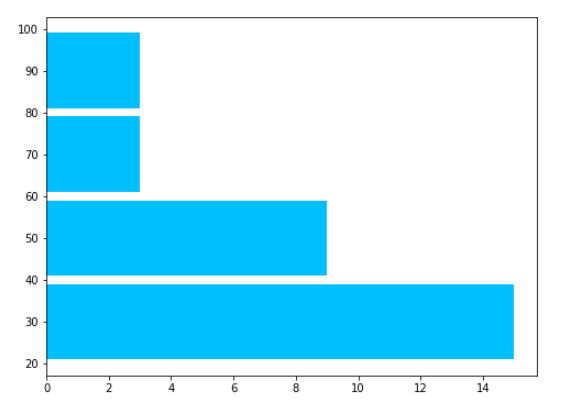#### Pro Tip: How to Improve Your Matplotlib Histogram

In addition to 1D histograms, you can also plot 2D histograms. To do so, you need values for both the x and y axis of the 2D histogram. The `hist2d` function is used to plot 2D histograms:

```stock_prices = [23,21,43,32,45,34,56,23,67,89]
years =[2009, 2010, 2011, 2012, 2013, 2014, 2015, 2016, 2017, 2018]

plt.hist2d(stock_prices, years)
plt.show()
```

This script plots the stock prices against their years as shown below: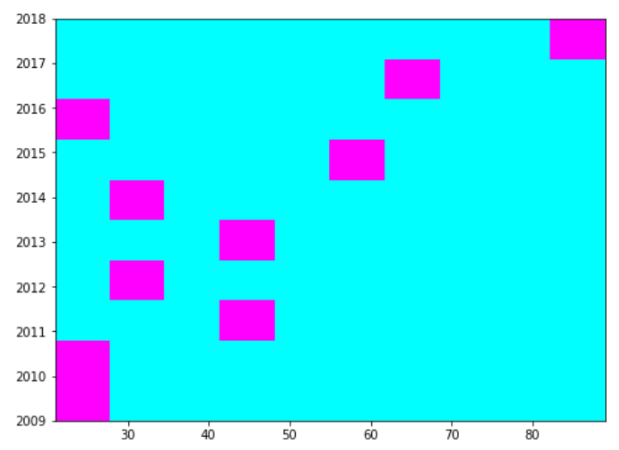### Hungry for More?

Here, we looked at a brief introduction to plotting data in Python with simple graphs like line plots and histograms, along with their variants. In the second part of this series, you’ll learn how to create bar plots, stack plots, scatter plots, and pie plots.

Want to learn more about Python for data science? Be sure to check out our Introduction to Python for Data Science online course for a beginner’s guide to Python programming.### Usman Malik

I am a machine learning and data science enthusiast with over 5 years of industry experience. Currently, I am pursuing my Ph.D. in machine learning. Python is my passion, machine learning is my hobby and data science is my profession.